Next: SRME versus HEMNO Up: 2-D Field Data Results Previous: Depth Migration Before and

## A closer look at the residuals

The theoretical development of the LSJIMP algorithm given in Chapterdefines the data and model residuals in mathematical and algorithmic terms, but in this section, I more practically illustrate the structure of and relationship between the residual vectors with a real data example. Figures-were computed at CMP 55 or 750 of the Mississippi Canyon 2-D dataset, in the sedimentary region of the data. Figures which display seismic data are divided in half along the time axis and clipped independently for viewing purposes.

Figureillustrates, as a function of conjugate gradient iteration, the norm of the data and model residuals from the LSJIMP inversion at CMP 55. Although the norm of the combined model and data residual [equation ()] is guaranteed to decrease with iteration, we see that the individual residuals may decrease at different rates, or even increase with iteration. Of particular interest in the Figure is the model residual corresponding to differentiation across individual images (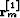in equation ()). As a starting guess, I simply spread'' a stacked trace of the primaries across all offsets, for all images.is roughly a measure of image dissimilarity. As the images are adjusted to fit variations in the data, the images become more dissimilar, and the model residual initially increases, before decreasing slowly with iteration.

 respow.gulf Figure 15 Individual data and model residuals as a function of iteration for LSJIMP inversion, CMP 55 of 750. rm_ord'', rm_off'', and rm_xtalk'' derive from,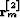, and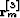in equation (), respectively, while rm_tot'' is the sum of these residuals. rd'' is the data residual, while total'' denotes the combined LSJIMP residual of equation ().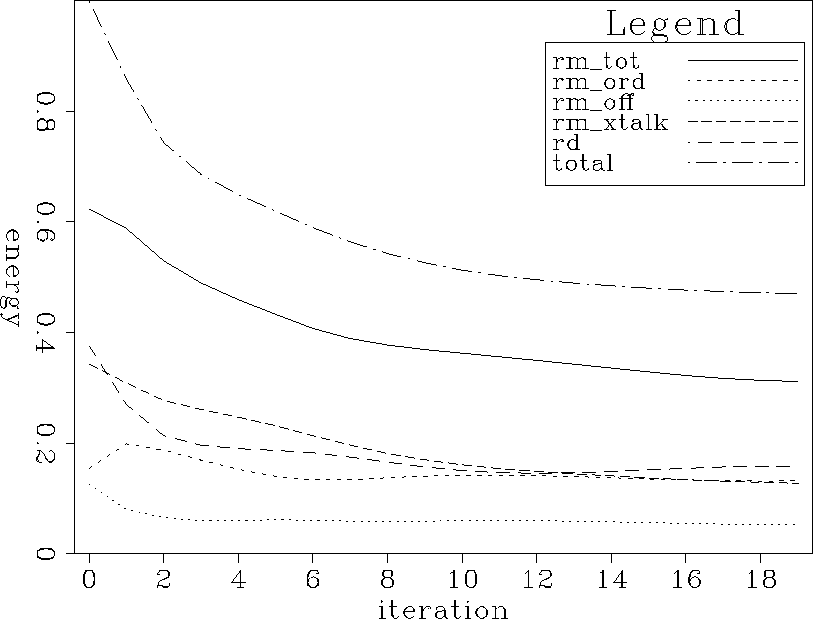Figurecompares the data residual and raw data at CMP 55. A somewhat similar comparison was made earlier, in Figures-, to examine the quality of fit to the multiples, but the earlier portions of the time axis were not shown. At earlier times, notice that the data residual contains some primary energy. The model regularization terms in the LSJIMP inversion cause the misfit. LSJIMP's working definition of signal'' includes events that are perfectly flat with offset on all images (with smooth'' AVO variation) and perfectly self-consistent multiple and primary images. If, for instance, the stacking velocity does not perfectly flatten a primary, or if primary and multiple events are misaligned or mis-modeled in terms of amplitude, we will see some primary energy in the data residual. Furthermore, notice increased misfit at near offsets versus far offsets. As noted in Section, the model regularization operators are not applied where the multiples provide no information - specifically, as far offsets, as dictated by Snell Resmpling. The fact that we see little to no residual primary energy at far offsets confirms the notion that model regularization terms cause the observed misfit at near offsets.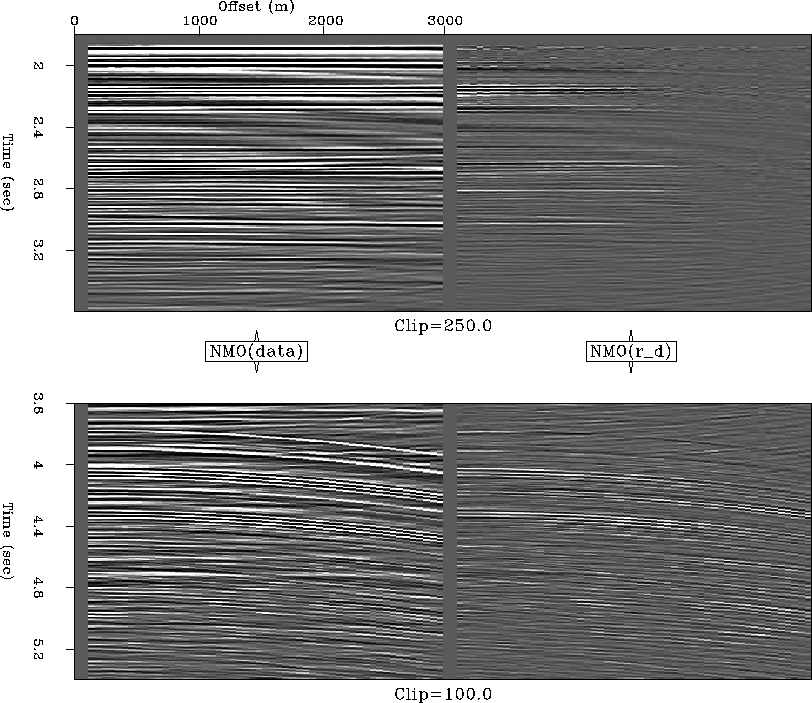resd.gulf
Figure 16
Raw data (left) and data residual (right) compared for CMP 55 of 750. NMO with primary stacking velocity applied to facilitate discrimination between multiples and primaries.

Figuresimply shows all nine (four multiple generators, first-order multiples only) panels of the model space at CMP 55. Comparing the primary image (''m0'') directly with the raw data shown in Figure, notice that obvious multiples have been strongly, but not totally, suppressed and that two prominent primary reflections between 4.4 and 4.8 seconds uncovered. This Figure and Figures-, which display the various model residual vectors, have the same graphical layout.model.gulf
Figure 17
LSJIMP model space at CMP 55 of 750. m0'' is the primary image,, while mikm'' corresponds to, the image of thesplit of the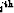-order pegleg multiple from themultiple generator.

Figureillustrates the model residual corresponding to the differencing across images regularization operator derived in Section, and toin equation (). First notice that the last panel, m114'' is blank. The differencing operator subtracts one image panel from the previous, in exactly the left-to-right order shown on the Figure. The difference is not defined for the last image, here m114''. The difference is zero-valued at far offsets, because the multiples provide no information here, as mentioned in the discussion of Figure. Above the onset of the seabed pure multiple, notice on the residual panels some primary energy, the presence of which can be explained by misalignment of primaries and multiples after imaging or by inaccuracies in the amplitude modeling of the multiples. In theory, after proper imaging, the multiples should be copies of the primary''; any deviations from this state will appear on the model residual shown in Figure. At later times, we notice considerable residual multiple energy. As discussed in Section(Figure), crosstalk events from one image panel to another do not generally coincide at far offsets.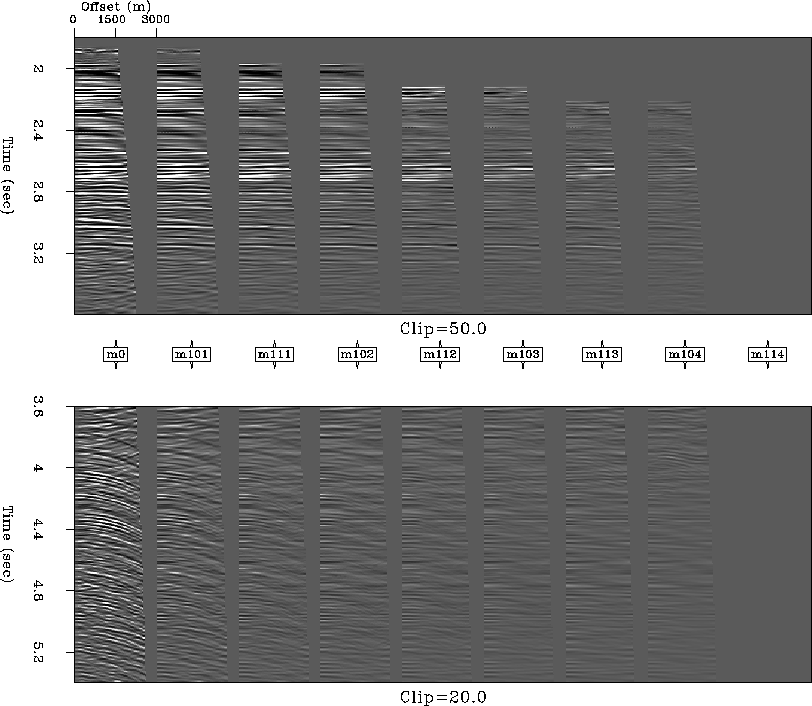resm.order.gulf
Figure 18
LSJIMP model residualat CMP 55 of 750. Each panel is the difference of one panel of the estimated model and the next panel to the right (see Figure).

Figureillustrates the model residual corresponding to the differencing across offset regularization operator derived in Section, and toin equation (). The panels are fairly simple to understand; the differencing filter amplifies high spatial wavenumber events, such as multiples at far offsets, random noise, or non-flat primaries. Notice that the difference is not taken at the far offsets of the multiple panels, where no multiple energy is recorded.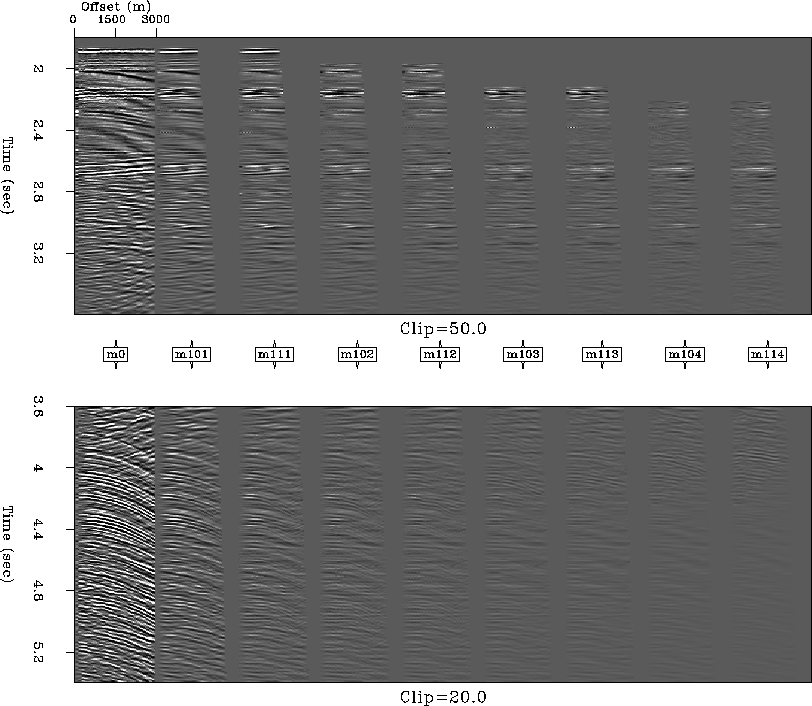resm.offsetx.gulf
Figure 19
LSJIMP model residualat CMP 55 of 750. Each sample of each panel is the difference of that sample and the adjacent sample in offset, on the corresponding estimated model panel (see Figure).

Figureillustrates the model residual corresponding to the crosstalk penalty weighting regularization operator derived in Section, and toin equation (). Conceptually, the panels are easily understood; each is simply the result of applying the crosstalk weight to the corresponding panel of the LSJIMP estimated model (Figure). In the case of the primary panel, m0'', the weight attempts to penalize all the modeled multiples. In the case of the multiple panels, the weight attempts to penalize multiples from all the other multiple generators.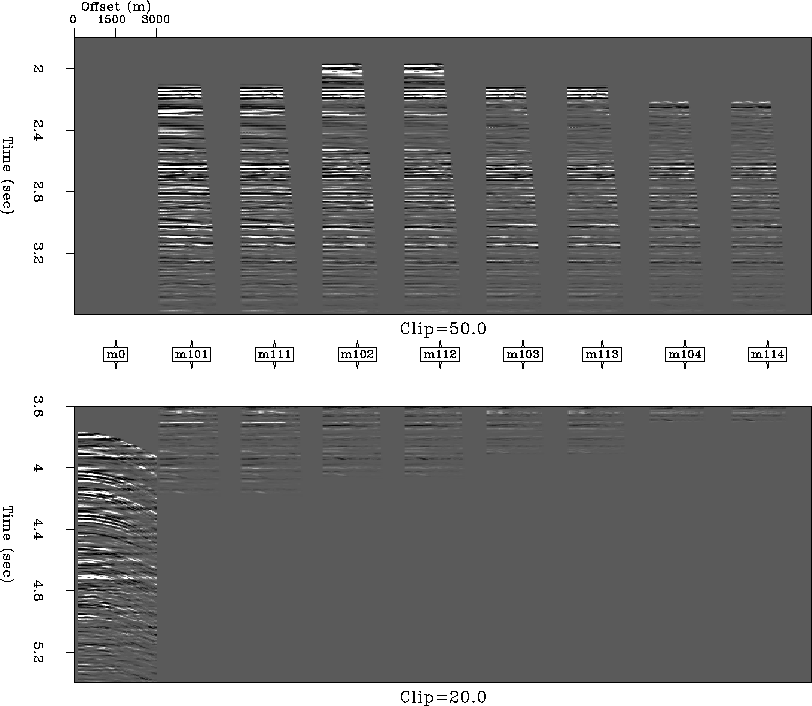resm.xtalk.gulf
Figure 20
LSJIMP model residualat CMP 55 of 750. Each panel is the result of applying crosstalk weights to the corresponding estimated model panel (see Figure).Next: SRME versus HEMNO Up: 2-D Field Data Results Previous: Depth Migration Before and
Stanford Exploration Project
5/30/2004